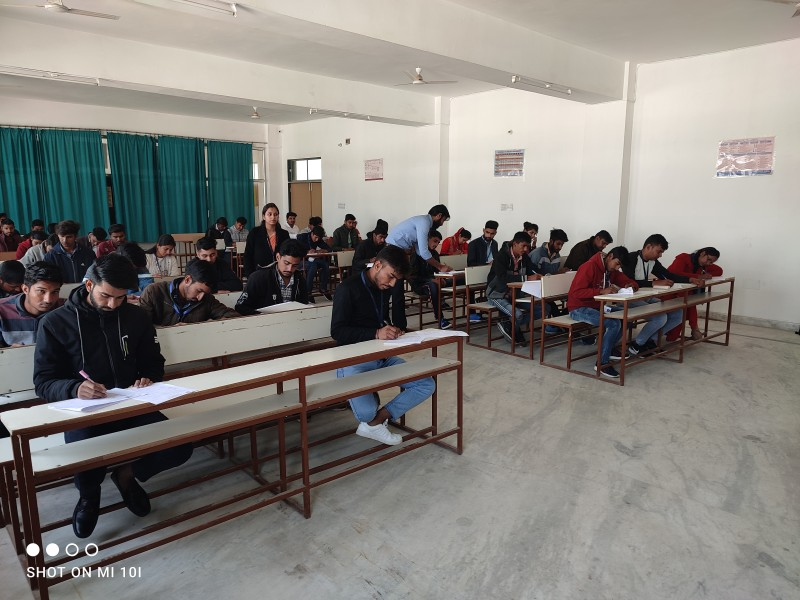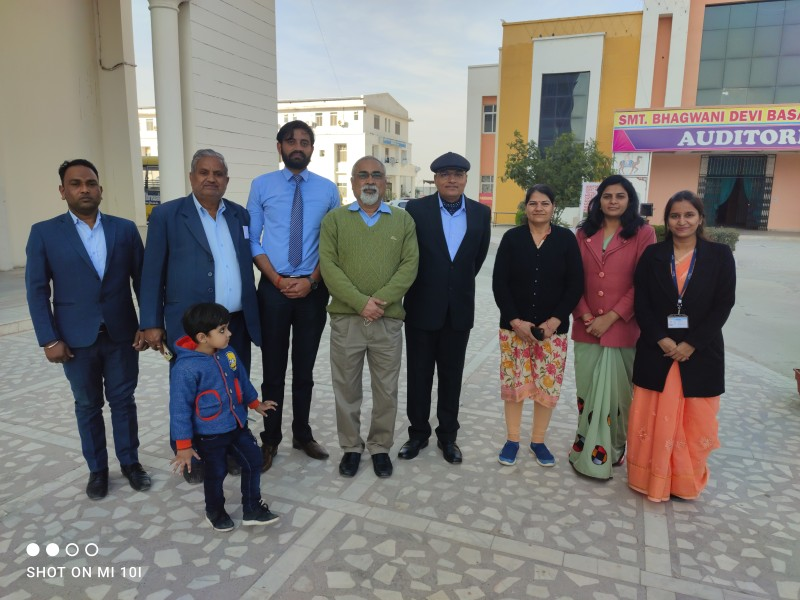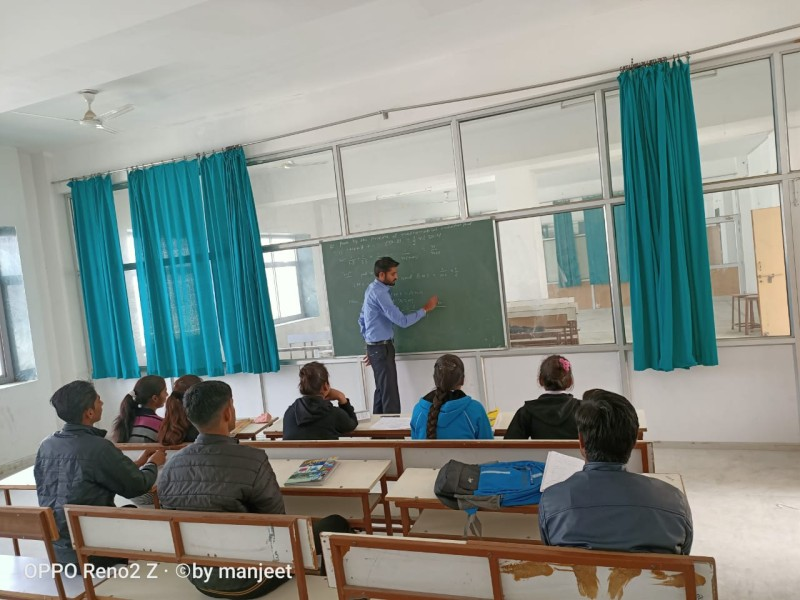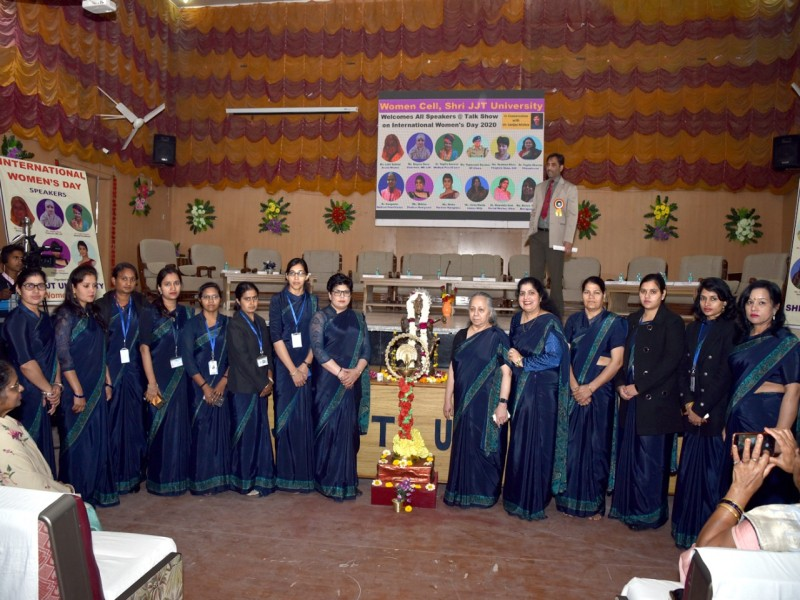## DEPARTMENT OF MATHEMATICS & STATISTICS

The Department of Mathematical Sciences was established with the objective of producing trained manpower for undertaking research and teaching in mathematics and allied branches of basic and applied science.

Faculty members of  the mathematics department are actively engaged in the major research areas as: Algebra, Analysis, Differential Equations, Fluid Dynamic, Fuzzy Mathematics, Industrial Statistics, Reliability, Probability Models, Health Statistics, Actuarial Science, Applied Statistics, and Computational Statistics.

Some of the main areas of research are listed below:
• Partial Differential Equations
• Numerical Analysis
• Numerical Linear Algebra
• Harmonic Analysis
• Topology
• Functional Analysis and Operator Theory
• Pseudo Differential Operator
• Algorithms and Graph Theory
• Optimization
• Game Theory
• Applied Probability
• Stochastic Models
• Financial Mathematics
• Dynamical Systems and Fractals
• Applied Statistics

COURSE TYPE COURSE NAME ELIGIBILITY CRITERIA DURATION (YEAR/SEM) REGISTRATION FEES FEE FOR GIRLS(ANNUAL/SEM) FEE FOR BOYS(ANNUAL/SEM)
B.Sc Bachelor Of Science 10+2(PCM)P.C.M 3 1000/- 3,000/- 12,000/-
M.Sc. Master Of Science B.Sc(PCM) 2 1000/- 6,250/- 25,000/-
Ph. D. Doctor Of Philosophy M.Sc in Mathematics 3 2000/- 60,000*/- 60,000*/-

Mathematcs Photo